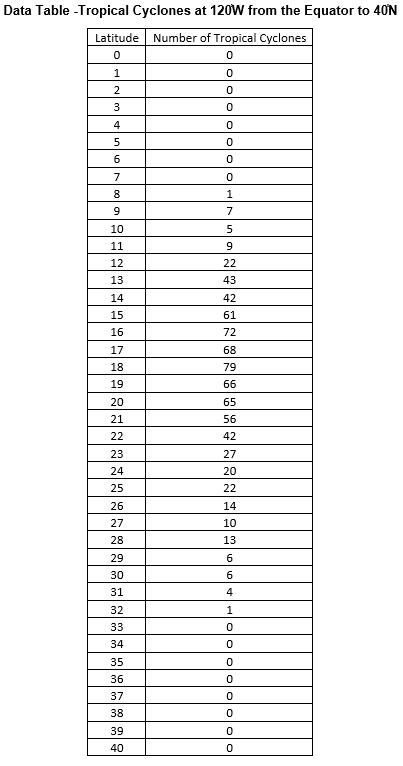## Tropical Cyclone Counts Create Scatter Plot

The purpose of this lesson is for students to create a scatter plot and evaluate the spread of the data. This is part of the Tropical Cyclone Counts Graphing Bundle and can be completed independently or with the other activities in the bundle.

### Mini Lesson

#### Review scatter plots briefly

1. A scatter plot is a type of graph which shows the relationship, or correlation, between two variables. One variable is on the x axis and the other is on the y axis.
2. The types of questions that might be answered with a scatter plot include:
• What is the relationship between variable # 1 and variable # 2?
• Does one variable go up when the other variable goes down?
• Does one variable go up when the other variable goes up?
• Is there a correlation between the two variables?
• Is there a positive, negative or no relationship between the variables?
• Scatter plots are very useful for understanding the correlation between two variables.

#### Plan your Scatter plot

1. What variable will be on the x-axis?
2. What variable will be on the y-axis?
3. What title will you use?
4. How many squares of the graph paper will you use for each unit on the x-axis?
5. How many squares of the graph paper will you use for each unit on the y-axis?

#### Create the Scatter plot Using the Answers to the Planning Questions

1. Draw and label the x-axis and the y-axis.
2. Label each axis.
3. Add the title.
4. Plot each point on the graph.
5. The plot will be analyzed in Mini Lesson 3 Question Set 1.